Polygon of Forces

Also found in: Dictionary.
The following article is from The Great Soviet Encyclopedia (1979). It might be outdated or ideologically biased.

Polygon of Forces

a broken line that is constructed to determine the resultant vector (geometric sum) of a given system of forces. In order to construct a force polygon for the system of forees F1, F2, … , Fn; (Figure 1, a), we successivel plot the following vectors in the chosen scale. The vector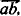which represents the force F1, is plotted from an arbitrary point a; the vector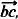which represents the force F2, is plotted from the head of the first vector. Continuing in this manner, we finally reach the vector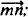this last vector, which represents the force Fn, (Figure 1,b), is plotted from the head m of the penulti-mate force.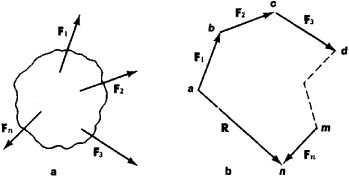Figure 1

The figure abcmn in Figure 1, b is called the polygon of forces, or force polygon. The vector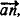which joins the tail of the first force with the head of the last force in the force polygon, represents the geometric sum R of the given system of forces. When the point n coincides with the point a, the force polygon is said to be closed. In this case, R = 0. The polygon law of forces can be derived by successive application of the parallelogram law of forces.

The construction of a force polygon is used in the graphical solution of problems in statics for systems offerees located in the same plane.